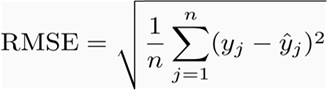2 views

I know I could implement a root mean squared error function like this:

def rmse(predictions, targets):

return np.sqrt(((predictions - targets) ** 2).mean())

What I'm looking for if this rmse function is implemented in a library somewhere, perhaps in scipy or scikit-learn?

closed

by (33.1k points)
edited by

Root mean squared error (RMSE): RMSE is a quadratic scoring rule that also measures the average magnitude of the error. It’s the square root of the average squared differences between prediction and actual observation.In the scikit learn library, sklearn.metrics has a mean_squared_error function. The RMSE is just the square root of values it returns.

For example:

from sklearn.metrics import mean_squared_error

from math import sqrt

rmse = sqrt(mean_squared_error(y_actual, y_predicted))

print(rmse)

If you want to learn K Means Clustering Algorithm then you can refer to the below video:

by (19.7k points)
Thanks, this solution helped!
by (19k points)
by (47.2k points)
This might work faster:

n = len(predictions)
rmse = np.linalg.norm(predictions - targets) / np.sqrt(n)
by (108k points)

sklearn.metrics has a mean_squared_error function. The RMSE is just the square root of whatever it returns.

from sklearn.metrics import mean_squared_error

from math import sqrt

rms = sqrt(mean_squared_error(y_actual, y_predicted))

by (32.1k points)
For me, this seemed to work. Thanks!
by (44.4k points)

There is no direct function which can be used, instead, you can find the Mean Squared Root and then Square Root it. Follow this example:

from sklearn.metrics import mean_squared_error

from math import sqrt

expected = [0.0, 0.5, 0.0, 0.5, 0.0]

predictions = [0.2, 0.4, 0.1, 0.6, 0.2]

mse = mean_squared_error(expected, predictions)

rmse = sqrt(mse)

print('RMSE: %f' % rmse)

by (29.3k points)
The mean square root and square root will be useful.
by (29.5k points)
by (19.9k points)

You could probably do it like this:

n = len(predictions)

rmse = np.linalg.norm(predictions - targets) / np.sqrt(n)

by (106k points)
edited by

​​​​​​No, there is not any library function for Root mean square error (RMSE) in python, but you can use the library Scikit Learn for machine learning and it can be easily employed by using Python language. It has the function for Mean Squared Error.

The function is named as mean_squared_error which  I am mentioning below, where y_true would be real class values for the data tuples and y_pred would be the predicted values, predicted by the machine learning algorithm you are using.

# The mean_squared_error(y_true, y_pred) function:-

You have to modify this function to get RMSE (by using sqrt function using Python).

# See the code below:-

from sklearn.metrics import mean_squared_error

from math import sqrt

RMSD = sqrt(mean_squared_error(testing_y, prediction))

print(RMSD)

+1 vote